# Simplification Questions for Competitive Exams

Simplification MCQ Questions for Competitive Exams. Objective Questions selected from the previous year Exam Question paper of SSC CGL, CPO, CHSL, GD, Police, Bank and UPSSSC. Type wise Question and Answer with solution and explanation.

## Simplification Questions : Type wise

### Type I : BODMAS (Bracket, Of, Division, Multiplication, Addition, Subtraction)

Q.1: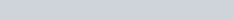$3 \frac 35 \times 3\frac 35 + 2 \times 3 \frac 35 \times \frac 25 + \frac 25 \times \frac 25 = ?$
a) 15
b) 16
c) 17
d) 18

Ans : b) 16
(a+b)2 = a2 + b2 +2ab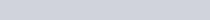$(3\frac 35 + \frac 25)^2 = (\frac {18+2} {5})^2 = 4^2 = 16$

Q. 2: The simplified value of the following is :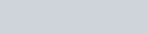$\dfrac{4}{15} of \dfrac{5}{8} \times 6 + 15 - 10$
a) 6
b) 3
c) 5
d) 4

Ans : a) 6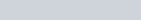$\frac{4}{15} of \frac{5}{8} \times 6 + 15 - 10$ =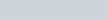$\frac{1}{6} \times 6 + 15 - 10$ =1+15-10 =6

Q.3: (0.9 × 0.9 × 0.9 + 0.1 × 0.1 × 0.1) is equal to
a) 0.73
b) 0.82
c) 0.91
d) 1.00

Ans : a) 0.73
(0.9)3 + (0.1)3 = 0.729 + 0.001 = 0.730

Q.4: The value of the following is
(0.98)3 + (0.02)3 + 3 x 0.98 x 0.02 -1
a) 1.98
b) 1.09
c) 1
d) 0

Ans : d) 0
(0.98)3 + (0.02)3 + 3 x 0.98 x 0.02 -1 = (0.98)3 + (0.02)3 + 3 x 0.98 x 0.02 (0.98 + 0.02) -1
= (0.98 + 0.02)3 -1 = 1-1 =0
(a+b)3 = a3 + b3 + 3ab (a+b)

Q.5: If * represent a number, than the value of * in following equation is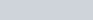$5 \frac 3* \times 3 \frac 12 = 19$
a) 7
b) 4
c) 6
d) 2

Ans : a) 7$5 \frac 3* \times 3 \frac 12 = 19$ =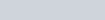$\frac {5 \times * + 3} {*} \times \frac72 = 19$
= 35 * +21 = 38 *
= 3 * = 21, * = 7

Q.6: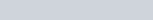$\frac {17}{15} \times \frac {17}{15} + \frac {2}{15} - \frac {17}{15} \times \frac {4}{15}$ is equal to
a) 0
b) 1
c) 10
d) 11

Ans : b) 1
(a – b)2 = a2 +b2 -2ab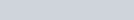$(\frac {17}{15} - \frac {2}{15}) ^2 = 1^2 =1$

Q.7: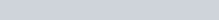$\frac {1}{30} + \frac {1}{42} + \frac {1}{56} + \frac {1}{72} + \frac {1}{90} + \frac {1}{110} = ?$
a)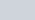$\sqrt {2} \frac {2}{27}$
b)$\frac 19$
c)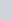$\frac {5}{27}$
d)$\frac{6}{55}$

Ans : d)$\frac{6}{55}$
=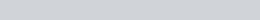$\frac{1}{5 \times 6} + \frac{1}{6 \times 7} + \frac{1}{7 \times 8} + \frac{1}{8 \times 9} + \frac{1}{9 \times 10} + \frac{1}{10 \times 11}$
=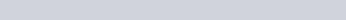$\frac{1}{5} - \frac{1}{6} + \frac{1}{6} - \frac{1}{7} + \frac{1}{7} - \frac{1}{8} + \frac{1}{8} - \frac{1}{9} + \frac{1}{9} - \frac{1}{10} + \frac{1}{10}- \frac{1}{11}$
=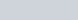$\frac 15 - \frac {1}{11} = \frac {6}{55}$

Q.8: 5-[4-{3-(3-3-6)}] is equal to
a) 10
b) 6
c) 4
d) 0

Ans : a) 10
5-[4-{3-(3-3-6)}] = 5-[4-{3-(-6)}] = 5-[4-{3+6}] = 5-[4-9] =5 -[-5] =10

### Type-II: Continued Fraction : Simplification Questions

Q.9: Find the value of$1- \dfrac{1}{1 + \dfrac {2}{3 +\dfrac{4}{5}}}$
a)$\frac {12}{29}$
b)$\frac {8}{19}$
c)$\frac {48}{29}$
d)$\frac {2}{19}$

Ans : c)$\frac {48}{29}$ [\toggle]

Q.10: If$2= x + \dfrac {1}{1+\dfrac {1}{3+\dfrac14}}$
than the value of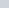$x$ is
a)$\frac {18}{17}$
b)$\frac {21}{17}$
c)$\frac {13}{17}$
d)$\frac {12}{17}$
[toggle] Ans : b)$\frac {21}{17}$

Simplification MCQ Questions for Competitive Exams

### Type – III: Square and Square Root : Simplification for Competitive Exams

Q.11: A number of boys raised ₹ 12544 for a famine fund, each boy has given as many rupees as there were boys. The number of boys was ?
a) 102
b) 112
c) 122
d) 132

Ans : b)112
Number of Boys x Amount Contributed = 12544 = 1122
Number of Boys = Amount Contributed = 112

Q.12: The sum of two numbers is 37 and the difference of their squares is 185, then the difference between the two numbers is :
a) 10
b) 4
c) 5
d) 3

Ans : c) 5
a+b=37, a2-b2 = 185
a2-b2 = (a-b) (a+b)
185 = (a-b) x 37
a-b = 185/37 =5

Q.13: The value of (11111)2 is
a) 12344321
b)121212121
c) 123454321
d) 11244311

Ans : c) 123454321
112 =121
1112 =12321
11112 = 1234321
111112 = 123454321

Q.14: The square root of following is :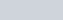$\frac {9.5 \times 0.085}{0.017 \times 0.019}$
a) 0.5
b) 5
c) 50
d) 500

Ans : c) 50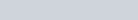$\frac {9.5 \times 0.085}{0.017 \times 0.019} = 50 \times 50$
Square Root is 50

Q.15: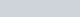$\sqrt{64} - \sqrt{36}$ is equal to :
a) -2
b) 2
c) 0
d) 1

Ans : b) 2
8-6=2

Q.16 : The sum of the squares of 3 consecutive positive numbers is 365. The sum of the numbers is :
a) 30
b) 33
c) 36
d) 45

Ans : b) 33
102+112+122 = 100+121+144 = 365
10+11+12 = 33

Q.17: Given that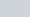$\sqrt {24}$ is approximately equal to 4.898. Then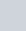$\sqrt \frac 83$ is nearly equal to :
a) 0.544
b) 1.333
c) 1.633
d) 2.666

Ans : c) 1.633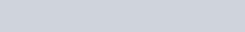$\sqrt \frac 83 = \sqrt \frac {8 \times 3}{3 \times 3} = \frac {\sqrt {24}}{3} = \frac {4.898}{3} = 1.633$

Q.18: The value of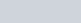$\frac {(75.8)^2 - (55.8)^2}{20}$
a) 20
b) 40
c) 121.6
d) 131.6

Ans : d) 131.6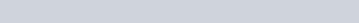$\frac {(75.8)^2 - (55.8)^2}{20} = \frac {(75.8 - 55.8) (75.8+55.8)}{20} = \frac {20 \times 131.6} {20} = 131.6$

Q.19: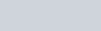$\sqrt { \frac {0.49}{0.25}} + \sqrt{\frac {0.81}{0.36} }$ is equal to :
a)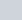$7 \frac {9}{10}$
b)$2 \frac {9}{10}$
c)$\frac {9}{10}$
d)$9 \frac {9}{10}$

Ans : b)$2 \frac {9}{10}$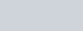$\sqrt { \frac {49}{25}} + \sqrt{\frac {81}{36} }$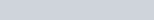$\frac75 +\frac 96 = \frac {87}{30} = \frac {29}{10} = 2\frac{9}{10}$

Q.20: When simplified, the product is equals to :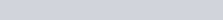$(2-\frac13) (2-\frac57) .............. (2-\frac {997}{999})$
a) $latex \frac {5}{999} b)$latex \frac {5}{3}
c) $latex \frac {1001}{999} d)$latex \frac {1001}{3}

Ans : d) \$latex \frac {1001}{3}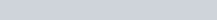$\frac 35 \times \frac 75 \times \frac 97 \times ........ \times \frac{1001}{999} = \frac{1001}{3}$

Simplification Objective Questions for Competitive Exams

### Type IV : Cube and Cube Root : Simplification

Q.21: What is the smallest number by which 625 must be divided so that the quotient is a perfect cube?
a) 25
b) 5
c) 2
d) 3

Ans : b) 5
625 = 5x5x5x5
Divide by 5 for cube.

Q.22: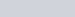$\sqrt {0.000125}$ is equal to
a) 0.5
b) 0.15
c) 0.05
d) 0.005

Ans : c) 0.05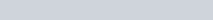$\sqrt {0.000125} = \sqrt {(0.05)^3} = 0.05$

Q.23: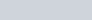$\sqrt{\sqrt{0.000064}}$ is equal to :
a) 0.0002
b) 0.02
c) 0.002
d) 0.2

Ans : d) 0.2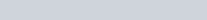$\sqrt{\sqrt{0.000064}} = \sqrt{0.008} = 0.2$

Q.24: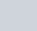$\sqrt{\frac{19}{513}}$ is equal to :
a)$\frac{1}{9}$
b)$\frac{1}{3}$
c)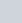$\frac{1}{ \sqrt{27}}$
d)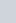$\frac{1}{\sqrt{3}}$

Ans : b)$\frac{1}{3}$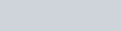$\sqrt{\frac{19}{513}} = \sqrt{\frac{1}{27}} = \frac13$

Q.25: The least possible value of A for which 90 × A is a perfect cube is :
a) 200
b) 300
c) 500
d) 600

Ans : b) 300
90 x A = 2x3x3x5xA
Therefore, A = 2x2x3x5x5 = 300

Q.26: The square of a natural number subtracted from its cube is 48. The number is :
a) 8
b) 6
c) 5
d) 4